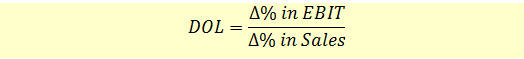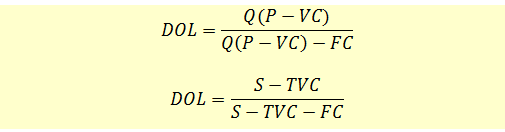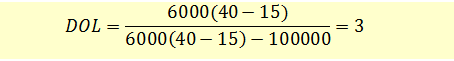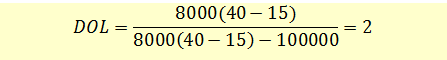# How to Calculate Degree of Operating Leverage

Operating leverage arises when a firm has fixed operating costs in its income stream. The presence of operating leverage magnifies the effects of changes in sales to the firm’s operating earnings, i.e., a change in the volume of sales results in a “more than proportional” change in operating profit (or loss).

The degree of operating leverage is a leverage ratio that measures how a percentage change in sales volume will affect the firm’s operating profits (EBIT), at a certain level of sales.We can calculate the DOL of a company at a particular level of sales as follows:Where:

• Q is the quantity of sales
• P is the price per unit
• VC is the variable cost
• FC is the fixed cost
• S is Sales
• TVC is total variable cost

### Example

Annie Davis wants to determine the degree of operating leverage at sales levels of 6,000 and 8,000 units.  Assume that:

Fixed costs are $100,000 Baskets are sold for$40 each

Variable costs are \$15 per basket

DOL at 6000 units:DOL at 8000 units:DOL is interpreted as follows: A 1% increase in sales above the 8,000 unit level increases EBIT by 2% because of the existing operating leverage of the firm.

Also observe that DOL is higher at lower level of sales and it declines as sales increase.